• dropna函数
万次阅读
2018-11-16 17:28:34

当想要删除缺失值大于几个的某行，而缺失值小于几个的那些行不删除，就可以用到 df.dropna(thresh=a)设置，其中，a是设定的几

更多相关内容
• Pandas是基于NumPy 的一种工具，该工具是为了解决数据分析任务而创建的。Pandas 纳入了大量库和一些标准的数据模型，提供了高效地操作大型数据集所需的...原文地址：Python pandas.DataFrame.dropna函数方法的使用 ...

Pandas是基于NumPy 的一种工具，该工具是为了解决数据分析任务而创建的。Pandas 纳入了大量库和一些标准的数据模型，提供了高效地操作大型数据集所需的工具。Pandas提供了大量能使我们快速便捷地处理数据的函数和方法。你很快就会发现，它是使Python成为强大而高效的数据分析环境的重要因素之一。本文主要介绍一下Pandas中pandas.DataFrame.dropna方法的使用。
原文地址：Python pandas.DataFrame.dropna函数方法的使用

展开全文•机器学习 sklearn 人工智能
• pandas数据清洗：drop函数、dropna函数、drop_duplicates函数详解1 drop函数简介1.1 构建学习数据1.2 删除行两种方法1.3 删除列两种方法2 dropna函数简介2.1 构建学习数据2.2 删除空值3种方法3 drop_duplicates函数...

# 1 drop函数简介

drop函数：用来删除数据表格中的列数据或行数据

df.drop(labels=None,axis=0
,index=None
,columns=None
,inplace=False)

参数简介
labels以列表形式赋值，待删除的行名或列名，与axis参数一起使用
axis确定删除行还是列，0为行，1为列
index以列表形式赋值，删除第几行；不与labels和axis参数连用
columns以列表形式赋值，删除第几列；不与labels和axis参数连用
inplace是否用新生成的列表替换原列表

## 1.1 构建学习数据

df = pd.DataFrame(np.arange(16).reshape(4, 4),
columns=['A', 'B', 'C', 'D'])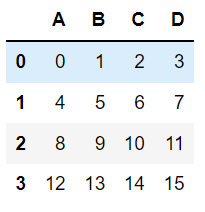## 1.2 删除行两种方法

方法一：使用index参数 []内是索引名，不是序号，要注意！
df.drop(index=[0,1],inplace=False)

方法二：使用labels和axis参数
df.drop(labels=[0,1],axis=0,inplace=False)

两者效果一样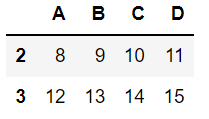## 1.3 删除列两种方法

方法一：使用columns参数
df.drop(columns=['A','B'],inplace=False)

方法二：使用labels和axis参数
df.drop(labels=['A','B'],axis=1,inplace=False)

两者效果一样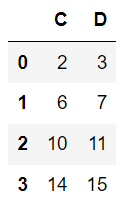# 2 dropna函数简介

dropna函数：用来删除数据表格中的空值数据

df.dropna(axis=0
,how='any'
,subset=None
,inplace=False)

参数简介
axis数据删除维度
howany：删除带有nan的行；all：删除全为nan的行
subset删除指定列空值数据
inplace是否用新生成的列表替换原列表

## 2.1 构建学习数据

df = pd.DataFrame({"name": ['Alfred', 'Batman', np.nan],
"toy": [np.nan, 'Batmobile', np.nan],
"born": [pd.NaT, pd.Timestamp("1940-04-25"),
pd.NaT]})
df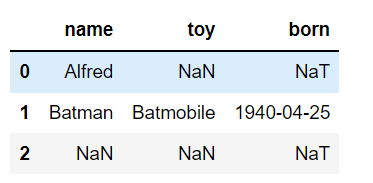## 2.2 删除空值3种方法

方法一：删除带空值的行
df.dropna(axis='index',how='any',inplace=False) #任何出现空值的行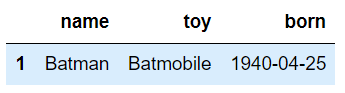方法二：删除带空值的列
df.dropna(axis='columns',how='all',inplace=False) # 全部为空值的列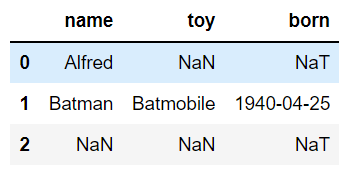方法三：删除指定列存在空值的行

方法三：删除指定列存在空值的行
df.dropna(axis='index',subset=['name'],how='any',inplace=False) # 仅删除name列存在空值的行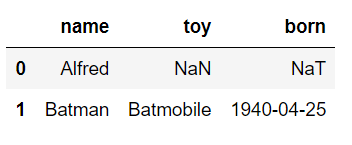# 3 drop_duplicates函数简介

drop_duplicates函数：用来去除数据表格中的重复数据

df.drop_duplicates(subset= None
,keep = 'first'
,inplace = False
)

参数简介
subset以列表形式赋值，需去重的列
keep“first”：仅保留第一次出现的重复行；“last”：仅保留最后一次出现的重复行；False：删除全部重复行
inplace是否用新生成的列表替换原列表

## 3.1 构建学习数据

import numpy as np
import pandas as pd
df = pd.DataFrame({'第一列':[1,1,3,4,5,3,4,5]
,'第二列':[1,1,20,20,30,40,50,50]
,'第三列':[1,1,20,20,200,600,500,600]
,'第四列':[1,1,'s','d','a','b','s','a']})
df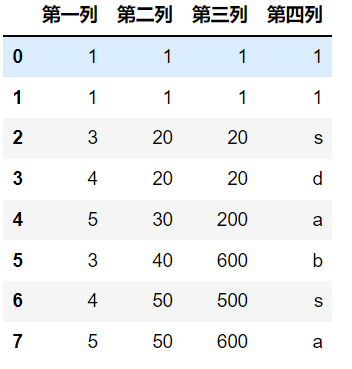## 3.2 去重方法

方法一：对整个表格，所有数据完全相同的行进行去重，并保留第一次出现的行
df.drop_duplicates(subset=None
,keep='first'
,inplace=False)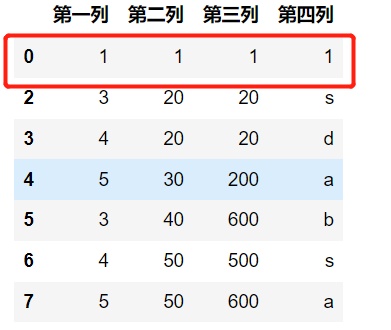方法二：删除所有重复的行
df.drop_duplicates(subset=None
,keep='first'
,inplace=False)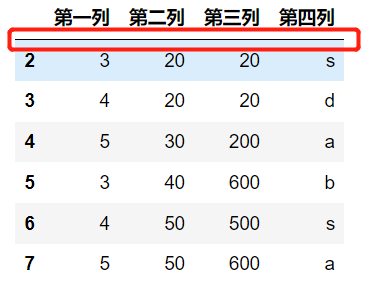方法三：对指定列进行去重
df.drop_duplicates(subset=['第二列','第三列']
,keep='first'
,inplace=False)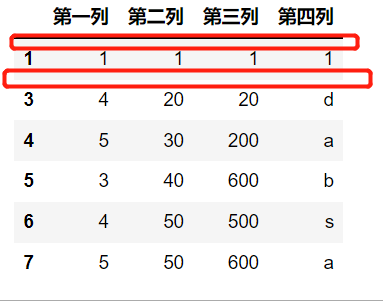## 3.3 reset_index函数重新设置索引

a = df.drop_duplicates(subset=['第二列'],keep=False,inplace=False)
a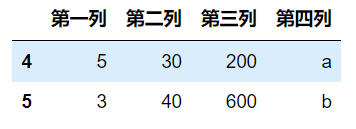不想保留原来的index，使用参数 drop=True
a.reset_index(drop=False)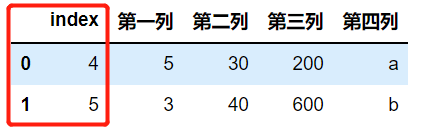以上就是pandas数据清洗，增删改查中删的主要内容，又成功了一大步！

================================================================下面点个赞👍，加个收藏⭐，方便下次使用啊！

展开全文python
• pandas使用dropna函数删除dataframe中全是缺失值的数据列（dropcolumns with all missing values in dataframe）数据挖掘 python 人工智能
• 函数介绍 函数举例 >>>df = pd.DataFrame({"name": ['Alfred', 'Batman', 'Catwoman'], ... "toy": [np.nan, 'Batmobile', 'Bullwhip'], ... "born": [pd.NaT, pd.Timest...
• pandas使用dropna函数删除dataframe中列缺失值的个数大于某一比例阈值的数据列（drop columns with missing values proportion more than a threshold in dataframe）数据挖掘 python 人工智能
• pandas使用dropna函数删除dataframe中列非缺失值的个数小于某一阈值的数据列（drop columns with non missing values less than a threshold in dataframe）python 数据挖掘 人工智能
• pandas使用dropna函数删除dataframe中列非缺失值的个数小于某一阈值数据列（dropcolumns with all missing values in dataframe）python 数据挖掘 人工智能
• pandas使用dropna函数删除dataframe中列缺失值的个数大于某一阈值的数据列（drop columns with missing values more than a threshold in dataframe） 目录python 数据挖掘 人工智能
• pandas使用dropna函数删除dataframe中所有包含缺失值的数据行（drop rows which contain missing vlaues in dataframe）python 数据挖掘 人工智能
• ## pandas 中 dropna()函数

万次阅读 2021-07-07 10:04:29
dropna()函数的作用是去除读入的数据中（DataFrame）含有NaN的行。 如下： >>> df = pd.DataFrame({"name": ['Alfred', 'Batman', 'Catwoman'], "toy": [np.nan, 'Batmobile', 'Bullwhip'], "born": [pd....python
• ## pandas dropna()函数

千次阅读 2022-02-11 15:56:44python 机器学习 数据挖掘
• 在用python处理数据时，我们通常会遇到数据不完整的情况，比如有空值的时候，我们想要删除控制所在的行或列，或者是我们给空值设置为某一个值。这时候就可以用dropna和fillna两个函数来实现空值的处理。python
• df2.dropna(axis = 0, subset = ['I071'] )  最终给的结果和报错是这样子的  [',I080,I071,I073,I074,I077,I130,I131,I140,I145,I146,I155,I157,I152,I160'] Traceback (most recent call last): ...python python
• 我使用了dropna()(它应该从数据帧中删除所有NA行)。然而，它不起作用。代码如下：import pandas as pdimport numpy as npprison_data = pd.read_csv('https://andrewshinsuke.me/docs/compas-scores-two-years.csv')...
• pd.dropna()函数 ● 选择题 以下关于dropna()函数说法错误的是： A 用于删除缺失数据 B axis=0：若某列有空值，则删除该列 C axis=1：若某列有空值，则删除该列 D how=all：该行/列全为空值，就删除该行/...python...